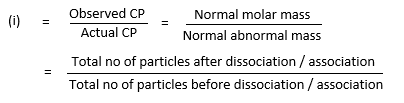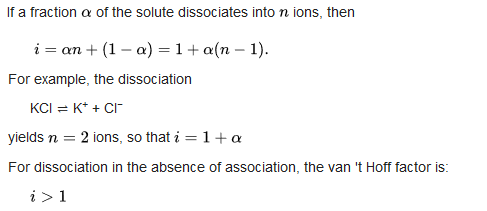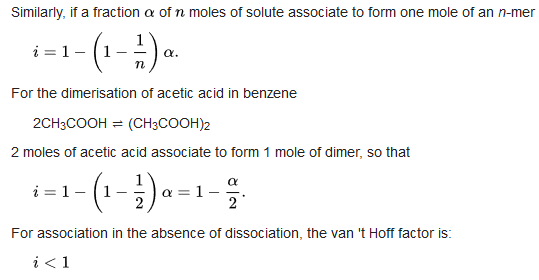Van't Hoff factor

It is the ratio between the actual concentration of particles produced and the concentration of a substance as calculated from its mass, when substance is dissolved.

• For most non-electrolytes dissolved in water, the van't Hoff factor is 1.

• For most ionic compounds dissolved in water, the van't Hoff factor is equal to the number of discrete ions in a formula unit of the substance.Dissociated solutes

The degree of dissociation is the fraction of the original solute molecules that have dissociated. It is usually indicated by the Greek symbol α.Associated solutes